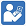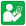Test Sheets - Multiply Fractions
Multiply Fractions - Test 1
Multiply Fractions - Test 2Practice Sheets - Multiply Fractions
Multiply Fractions - Practice 1
Multiply Fractions - Practice 2
Multiply Fractions - Practice 3